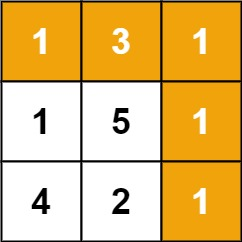V1

2022/10/10阅读：57主题：蔷薇紫

1. 动态规划基础知识
2. 单序列问题
3. 双序列问题
4. 矩阵路径问题
5. 背包问题

# 动态规划基础知识

• 如果要求列举出所有的解决，那选择用回溯法解决
• 如果求一个问题的最优解(`最大值或者最小值`)，或者求问题的数目，那选择动态规划

1. 采用递归的代码按照从上往下的顺序求解，那么每求出一个小问题的解就缓存下来，这样下次再遇到相同的小问题就不用重复计算。
2. 按照从下往上的顺序，从解决最小的问题开始，并把已经解决的小问题的解存储下来（大部分都是存储在一维数组或者二维数组中），然后把小问题的解组合起来逐步解决大问题。

## 爬楼梯的最小成本

### 分析

1. 爬上一个有多级台阶的楼梯需要若干步，每一步有两个选择
• 既可以往上爬1级台阶，
• 也可以爬2级台阶
2. 计算爬上楼梯最少成本，而不是所有的解 -- 抛弃回溯法，选择动态规划

### 确定状态转移方程

`f(i)`表示从楼梯的第`i`级台阶再往上爬的最少成本。如果一个楼梯有`n`级台阶(台阶从`0`开始计数，从第`0`级一直到第`n-1`级)，由于一次可以爬1级或2级台阶，因此可以从第`n-2`级台阶或第`n-1`级台阶爬到楼梯的顶部，`f(n-1)``f(n-2)`的最小值就是这个问题的最优解

• 既可以从第`i-1`级台阶爬上第`i`级台阶，
• 也可以从第`i-2`级台阶爬上第`i`级台阶。

• 如果`i`等于0,可以直接从第`0`级台阶往上爬 -> `f(0) = cost`
• 如果`i`等于1，可以直接从第`1`级台阶往上爬 -> `f(1) = cost`

### 代码实现

#### 递归代码

``function minCost(cost){  let len = cost.length;  return Math.min(helper(cost,len -2),helper(cost,len -1));}``

``function helper(cost,i){  if(i<2){ // 基线条件    return cost[i]  }  return Math.min(helper(cost,i-2),helper(cost,i-1)) + cost[i];}``

1. 递归函数`helper`和状态转移方程相对应
2. 求解`f(i)`这个问题的解，依赖于求解`f(i-1)``f(i-2)`这两个子问题的解，由于求解`f(i-1)``f(i-2)`这两个子问题有重叠的部分。如果只是简单的将状态转移方程转换成递归的代码就会带来严重的效率问题

#### 使用缓存的递归代码

``function minCost(cost){  let len = cost.length;  if(len<=2){    return Math.min(cost,cost)  }  //初始化都为 0 计算之后应该是大于 0 的结果  let dp = new Array(len).fill(0);   //从最上层的台阶往下走 从上到下进入递归  helper(cost,len -1,dp);  return Math.min(dp[len-2],dp[len-1]);}``

``function helper(cost,i,dp){  if(i<2){ //基线条件    dp[i] = cost[i]  }else if(dp[i]==0){    helper(cost,i-2,dp);    helper(cost,i-1,dp);    dp[i] = Math.min(dp[i-2],dp[i-1]) + cost[i]  }  }``

1. 数组`dp`用来保存求解每个问题结果的缓存
• `dp[i]`用来保存`f(i)`的计算结果
• 该数组的每个元素都初始化为`0` -> `new Array(len).fill(0)`
2. 由于从每级台阶往上爬的成本都是正数，如果某个问题`f(i)`之前已经求解过，那么`dp[i]`的缓存的结果将是一个大于0的数值。
• 只有当`dp[i]`等于0时，它对应的`f(i)`之前还没有被求解过
3. 有了缓存`dp`，就能确保每个问题`f(i)`只需要求解一次。
4. 在辅助函数中，针对`i<2`的情况，是直接返回`dp[i] = cost[i]`,但是，没有处理比较特殊的情况
• `cost.length ≤2`时，需要做一次特殊处理。
• 直接返回它们的最小值即可 ` Maht.min(cost,cost)`

#### 空间复杂度为O(n)的迭代代码

``function minCost(cost){  let len = cost.length;  let dp = new Array(len).fill(0);  dp = cost;  dp = cost;    for(let i =2;i<len;i++){    dp[i] = Math.min(dp[i-2],dp[i-1]) + cost[i]  }  return Math.min(dp[len-2],dp[len-1])}``

1. 先求得`f(0)``f(1)`的结果并保存到数组`dp`前两个位置
• `dp = cost;`
• `dp = cost;`
2. 用一个`for`循环根据状态转移方程逐一求解`f(2)``f(n-1)`
3. 时间复杂度和空间复杂度都是`O(n)`

#### 空间复杂度为O(1)的迭代代码

``function minCost(cost){  let len = cost.length;  let dp = [cost,cost];    fort(let i =2;i<len;i++){    dp[i&1] = Math.min(dp,dp)+cost[i]  }  return Math.min(dp,dp);}``

1. `dp`的长度是2，求解的`f(i)`的结果保存在数组下标为`i&1`的位置。
2. 可以根据`f(i-1)``f(i-2)`的结果计算出`f(i)`的结果，并`f(i)`的结果写入之前保存`f(i-2)`的位置
• `f(i)`的结果覆盖`f(i-2)`的结果并不会带来任何问题
• 因为，接下来求解`f(i+1)`只需要`f(i)`的结果和`f(i-1)`的结果
• 不需要`f(i-2)`的结果

#### 比较4种解法

1. 第一种解法在找出状态转移方程之后直接将其准换成递归代码，由于计算过程中存在大量的重复计算，时间复杂度很大
2. 第二种解法在第一种解法的基础上添加了一个一位数组，用来缓存已经求解的结果。
• 有了这个长度`O(n)`的数据，缓存之后就能够确保每个子问题值需要计算一次
• 时间复杂度为`O(n)`
3. 第三种解法时间复杂度和空间复杂度都是`O(n)`。和第二种解法有两方面的不同
1. 求解顺序不同： 第二种解法从大的子问题出发，采用自上而下的顺序求解；而第三种解法从子问题出发，采用自下而上的顺序求解。
2. 代码实现思路不同：第二种采用递归方式实现；而第三种采用迭代方式实现。
4. 第四种解法在第三种解法的基础上进一步优化空间效率，使空间下来变成`O(1)`

# 单序列问题

## 房屋偷盗

### 分析

1. 应用动态规划解决问题的关键就是在于找出转移方程
2. 用动态规划解决单序列的问题的关键在于找到序列中一个元素对应的解和前面若干元素对应的解的关系，并用状态转移方程表示。
3. 假设街道上有`n`幢房屋（分别用`0~n-1`标号），小偷从标号为`0`的房屋开始偷东西。
• `f(i)`表示小偷从标号为`0`的房屋开始标号为`i`的房屋为止最多能偷取到的财物最大值
• `f(n-1)`的值是小偷从`n`幢房屋中能偷取的最多财物的数量。
4. 小偷在标号为`i`的房屋前有两个选择
1. 选择进去偷东西 - 由于有报警系统，因此他不能进入相邻的标号为`i-1`的房屋内，之前他最多能偷取的财物的最大值是`f(i-2)`,因此，如果进入标号为`i`的房屋并进行偷盗，他最多能偷的`f(i-2)+nums[i]`
2. 不进入标号为`i`的房屋 - 那么他可以进入标号为`i-1`的房屋，因为此时他最多能偷取的财物数量为`f(i-1)`
5. 在到达标号为`i`的房屋时，他能偷取的财物的最大值就是两个选项的最大值
• `f(i) = max(f(i-2)+nums[i],f(i-1))`
6. 状态转移方程还有一个隐含条件，即`i`大于或等于2
• `i`等于0时，`f(0) = nums`
• `i`等于1时，`f(1)= max(nums,nums)`

### 带缓存的递归代码

``function rot(nums){  if(nums.length==0) return 0;    let dp = new Array(nums.length).fill(-1);  (function helper(nums,i,dp){    if(i ==0){      dp[i] = nums    }else if(i ==1){      dp[i] = Math.max(nums,nums)    }else if(dp[i]<0){      helper(nums,i -2,dp);      helper(nums,i -1,dp);      dp[i] = Math.max(dp[i-1],dp[i-2]+nums[i])    }  })(nums,nums.length-1,dp);  return dp[nums.length-1]}``

1. 函数`helper`就是将状态转移方程`f(i)= max(f(i-2)+nums[i],f(i-1))`翻译成js的代码。
2. 状态转移方程要求`i`大于或等于`2`，因此函数`helper`单独处理了`i`分别等于`0``1`的特殊情况

### 空间复杂度为O(n)的迭代代码

• 然后用`f(0)``f(1)`的值求出`f(2)`
• `f(1)``f(2)`的值求出`f(3)`
• 依次类推，直至求出`f(n-1)`
``function rob(nums){  if(nums.length==0) return 0;    let dp = new Array(nums.length).fill(0);  dp = nums;  if(nums.length>1){    dp = Math.max(nums,nums)  }    for(let i=2;i<nums.length;i++){    dp[i] = Math.max(dp[i-1],dp[i-2]+nums[i])  }  return dp[nums.length-1]}``

### 空间复杂度为O(1)的迭代代码

``function rob(nums){  if(nums.length==0) return 0;  let dp = new Array(2).fill(0);  dp = nums;    if(nums.length>1){    dp = Math.max(nums,nums)  }    for(let i=2;i<nums.length;i++){    dp[i&1] = Math.max(dp[(i-1)&1],dp[(i-2)&1]+nums[i])  }  return dp[(nums.length-1)&1]}``

1. 数组`dp`的长度为`2`，将`f(i)`的计算结果保存在数组下标为`dp[i&1]`的位置
• `f(i)``f(i-2)`将保存到数组的同一个位置
2. 根据`f(i-1)``f(i-2)`的结果计算出`f(i)`,然后用`f(i)`的结果写入数组原来保存`f(i-2)`的位置。
3. 接下来用`f(-1)``f(i)`的结果计算出`f(i+1)`

## 环形房屋偷盗

### 分析

1. 线性街道上的房屋和环形街道上的房屋存在不同之处
2. 如果`n`幢房屋围成一个首尾相接的环形，那么标号为`0`的房屋和标号为`n-1`的房屋相邻。如果小偷进入这两幢房屋内偷东西就会触发报警系统。
3. 这个问题和线性街道的区别在于小偷不能同时到标号为`0``n-1`的两幢房屋内偷东西
4. 因此将这个问题分解成两个子问题
1. 求从标号为`0`开始到标号为`n-2`结束的房屋内偷得的最多财物的数量
2. 求从标号为`1`开始到标号为`n-1`结束的房屋内偷得的最多财物的数量

### 代码实现

``function rob(nums){  if(nums.length ==0) return 0;  if(nums.length ==1) return nums;    let result1 = helper(nums,0,nums.length -2);  let result2 = helper(nums,1,nums.length -1);  return Math.max(result1,result2)}``

``function helper(nums,start,end){  let dp = new Array(2).fill(0);  dp = nums[start];  if(start<end){    dp = Math.max(nums[start],nums[start+1])  }  // 注意i的取值  for(let i= start+2;i<=end;i++){    let j = i - start; //这里是关键    dp[j&1] = Math.max(dp[(j-1)&1],dp[(j-2)&1]+nums[i])  }  // 最后取值  return dp[(end- start)&1]}``

# 双序列问题

• `f(i,j)`
• 定义第一个序列中下标从`0``i`的子序列
• 和第二个序列中下标从`0``j`的子序列

1. `f(i-1,j-1)`
2. `f(i-1,j)`
3. `f(i,j-1)`

## 最长公共子序列

### 分析确定状态转移方程

1. 应用动态规划解决问题的关键在于确定状态转移方程。
2. 由于输入有两个字符串，因此状态转移方程有两个参数。
• 用函数`f(i,j)`表示
• 第1个字符串中下标从`0``i`的字符串(记为`s1[0..i]`)
• 第2个字符串中下标从`0``j`的字符串(记为`s2[0..j]`)
• 的最长公共序列的长度
3. 如果第1个字符串的长度是`m`，第2个字符串的长度是`n`，那么`f(m-1,n-1)`就是问题的解
4. 如果第1个字符串中下标为`i`的字符(记为`s1[i]`)与第2个字符串中下标为`j`(记为`s2[j]`)的字符相同
• 那么`f(i,j)`相当于在`s1[0..i-1]``s2[0..j-1]`的最长公共子序列的后面添加一个公共字符
• 也就是`f(i,j) = f(i-1,j-1)+1`
5. 如果字符`s1[i]`与字符`s2[j]`不相同，则这两个字符不可能同时出现在`s1[0..i]``s2[0..j]`的公共子序列中。此时`s1[0..i]``s2[0..j]`的最长公共子序列，
• 要么是`s1[0..i-1]``s2[0..j]`的最长公共子序列
• 要么是`s1[0..i]``s2[0..j-1]`的最长公共子序列
• 也就是，此时`f(i,j)``f(i-1,j)``f(i,j-1)`最大值
6. 那么状态转移方程为
• `s1[i]==s2[j]`, `f(i,j) = f(i-1,j-1)+1`
• `s1[i]!=s2[j]`, `f(i,j) = max(f(i-1,j),f(i,j-1))`
7. 上述状态转移方程的`i`或者`j`等于`0`时，即求`f(0,j)``f(i,0)`时可能需要的`f(-1,j)``f(i,-1)`的值。
• `f(0,j)`的含义是`s1[0..0]``s2[0..j]`这两个字符串的最长公共子序列的长度
• 即第1个字符串只包含一个下标为`0`的字符，那么`f(-1,j)`对应的第1个子字符串再减少一个字符
• 所以第1个字符串是空字符串
• 任意空字符串和另一个字符串的公共子序列的长度都是`0`,所以`f(-1,j)`的值等于0

### 根据状态转移方程写代码

1. 先将表格中`i`等于`-1`对应的行和`j`等于`-1`对应的列都初始化为`0`
2. 然后按照从上到下、从左到右的顺序填充表格中的其他位置

``function longestCommonSubsequence(s1,s2){  let l1 = s1.length;  let l2 = s2.length;  // 注意行、列的长度  （l1+1/l2+1）  let dp = new Array(l1+1).fill(0)              .map(()=>                new Array(l2+1).fill(0)               )    for(let i=0;i<l1;i++){    for(let j=0;j<l2;j++){      if(s1[i]==s2[j]){        dp[i+1][j+1]= dp[i][j]+1      }else {        dp[i+1][j+1] = Math.max(dp[i][j+1],dp[i+1][j])      }    }  }  return dp[l1][l2];}``

1. 由于表格中有`i`等于`-1`对应的行和`j`等于`-1`对应的列，因此如果输入字符串的长度分别为`m``n`，那么代码中的二维数组`dp`的行数和列数分别是`m+1``n+1`
• `f(i,j)`的值保存在`dp[i+1][j+1]`

### 优化空间效率，只保存表格的两行

`f(i,j)`的值依赖于表格中

• 左上角`f(i-1,j-1)`的值、
• 正上方`f(i-1,j)`的值
• 同一行左边`f(i,j-1)`的值

``function longestCommonSubsequence(s1,s2){  let l1 = s1.length;  let l2 = s2.length;  if(l1<l2){    return longestCommonSubsequence(s2,s1)  }  //行数为2  let dp = new Array(2).fill(0)            .map(()=>              new Array(l2+1).fill(0)              )    for(let i=0;i<l1;i++){    for(let j=0;j<l2;j++){      if(s1[i]==s2[j]){        // 处理行数        dp[(i+1)&1][j+1]= dp[i&1][j]+1;      }else {        // 处理行数        dp[(i+1)&1][j+1] = Math.max(                              dp[i&1][j+1],                              dp[(i+1)&1][j]                            )      }    }  }  return dp[l1&1][l2]}``

1. 二维数组`dp`只有两行，`f(i,j)`的值保存在`dp[(i+1)&1][j+1]`中。
2. 由于数组`dp`的行数是一个常数，因此此时的空间复杂度是`O(min(m,n))`

### 进一步优化空间效率，只需要一个一维数组

• 一维数组的每个位置需要保存原来表格中上下两格的信息
• `f(i,j)``f(i-1,j)`都保存在数组`dp`下标`j+1`的位置。

``function longestCommonSubsequence(s1,s2){  let l1 = s1.length;  let l2 = s2.length;  if(l1<l2){    return longestCommonSubsequence(s2,s1)  }    let dp = new Array(l2+1).fill(0);  for(let i=0;i<l1;i++){    let prev = dp;    for(let j = 0;j<l2;j++){      let cur ;      if(s1[i]==s2[j]){        cur = prev +1;      }else {        cur = Math.max(dp[j],dp[j+1])      }      prev = dp[j+1];      dp[j+1]= cur;    }  }  return dp[l2]}``

1. 变量`prev`用来保存数组中被替换的值。
• 在计算`f(i,j)`之前，变量`prev`保存的是`f(i-1,j-1)`的值。
• 在计算`f(i,j)`（代码中变量`cur`）之后，将它保存到`dp[j+1]`中。
2. 在保存`f(i,j)`之前，将保存在`dp[j+1]`中的值(即`f(i-1,j)`)临时保存到变量`prev`
3. 下一步计算`f(i,j+1)`时可以从变量`prev`中得到`f(i-1,j)`
4. 在代码`cur = Math.max(dp[j],dp[j+1])`
• `dp[j]`对应的是`f(i,j-1)`
• `dp[j+1]`对应的是`f(i-1,j)`
5. 由于是按照从上而下、从左到右的顺序填充表格，因此在计算`f(i,j)`之前，`f(i,j-1)`的值已经计算出来并保存到`dp[j]`的位置
• 此时`f(i,j)`的值还没有计算出来，因此保存在`dp[j+1]`中的还是`f(i-1,j)`的值

# 矩阵路径问题

• 左上角`f(i-1,j-1)`的值、
• 正上方`f(i-1,j)`的值
• 同一行左边`f(i,j-1)`的值

## 路径的数目

1. 向右 -> 向下 -> 向下
2. 向下 -> 向下 -> 向右
3. 向下 -> 向右 -> 向下

• 要么向下走，
• 要么向右走。

### 分析确定状态转移方程

1. 用函数`f(i,j)`表示从格子的左上角坐标为`(0,0)`的位置出发到达坐标为`(i,j)`的位置的路径数目
• 如果格子的大小为`m×n`,那么`f(m-1,n-1)`就是问题的解
2. `i`等于0时，机器人位于格子最上面的一行，机器人不可能从某个位置向下走一步到达一个行号`i`等于0的位置。
• 因此，`f(0,j)`等于1
• 即机器人只有一种方法可以到达坐标为`f(0,j)`的位置
• `f(0,j-1)`的位置向右走一步
3. `j`等于0时，机器人位于格子最左边的一列，机器人不可能从某个位置向右走一步到达一个列号`j`为0的位置。
• 因此，`f(i,0)`等于1
• 即机器人只有一种方法可以到达坐标为`(i,0)`的位置
• `(i-1,0)`的位置向下走一步
4. 当行号`i`、列号`j`都大于0时，机器人有两种方法可以到达坐标为`(i,j)`的位置。
• 可以从坐标为`(i-1,j)`的位置向下走一步
• 可以从坐标为`(i,j-1)`的位置向右走一步
• 因此，`f(i,j)= f(i-1,j)+f(i,j-1)`

### 根据状态转移方程写递归代码

``function uniquePaths(m,n){  let dp = new Array(m).fill(0)              .map(()=>                  new Array(n).fill(0)                )  return (function helper(i,j,dp){    if(dp[i][j]==0){      if(i==0||j==0){        dp[i][j] =1;      }else {        dp[i][j] = helper(i-1,j,dp) + helper(i,j-1,dp)      }    }    return dp[i][j]  })(m-1,n-1,dp)}``

1. 为了避免不必要的重复计算，需要用一个二维数组缓存`f(i,j)`的结果
2. `f(i,j)`保存在`dp[i][j]`

### 迭代代码

• `f(0,j)``f(i,0)`的值都等于1，将表格的第1行和第1列的值都设为1
• 计算第2行(行号为1)剩下的位置的值
• 按照状态转移方程,`f(1,1)`等于`f(0,1)``f(1,0)`之和
• `f(1,2)`等于`f(1,1)``f(0,2)`之和
• 依次类推，计算剩余行数
``function uniquePaths(m,n){ let dp = new Array(m).fill(0).map((item,index)=>{        if(index == 0){            // 初始化f(0,j)             return new Array(n).fill(1)        }else {            return new Array(n).fill(0)        }  });    for(let i=1;i<m;i++){    dp[i] =1  }    for(let i=1;i<m;i++){    for(let j=1;j<n;j++){      dp[i][j] = dp[i][j-1]+dp[i-1][j]    }  }  return dp[m-1][n-1]}``

### 优化空间效率

• 这个位置在计算`f(i,j)`之前保存的是`f(i-1,j)`的值
• 计算`f(i,j)`之后，保存的是`f(i,j)`的值

``function uniquePaths(m,n){  // 数组长度为列数  let dp = new Array(n).fill(1);    for(let i=1;i<m;i++){    for(let j=1;j<n;j++){      dp[j] += dp[j-1]    }  }  return dp[n-1]}``

1. `dp`是一个一维数组，`f(i-1,j)``f(i,j)`都保存在`dp[j]`中。
2. 仍然用一个二重循环按照状态转移方程计算
3. 循环体内的`dp[j]+=dp[j-1]`可以看成`dp[j]= dp[j]+dp[j-1]`
• 在赋值运算符的右边 `dp[j]`保存的是`f(i-1,j)``dp[j-1]`中保存的是`f(i,j-1)`
• 计算`f(i,j)`之前，按照从左到右的顺序`f(i,j-1)`的值已经计算出来并保存在`dp[j-1]`
• `f(i-1,j)``f(i,j-1)`的值计算出`f(i,j)`之后将结果保存到`dp[j]`
4. 虽然之前保存在`dp[j]`中的`f(i-1,j)`的值被覆盖，但这个值不在需要，因此覆盖这个值并不会出现任何问题

## 最小路径之和输入：`grid = [[1,3,1],[1,5,1],[4,2,1]]`

• 要么向下走，
• 要么向右走。

### 分析确定状态转移方程

1. 用函数`f(i,j)`表示从格子的左上角坐标为`(0,0)`的位置(用`grid`表示)出发到达坐标为`(i,j)`的位置(用`grid[i][j]`表示)的路径的数字之和的最小值
2. 如果格子的大小为`m x n`,那么`f(m-1,n-1)`就是问题的解
3. `i`等于0时，机器人位于格子的最上面的一行，机器人不可能从某个位置向下走一步到达一个行号`i`等于0的位置。
• 此时只有一条从左到右的路径，因此`f(0,j)`最上面一行从`grid`开始到`grid[j]`为止所有格子的值之和
4. `j`等于0时，机器人位于格子的最左边的一列，机器人不可能从某个位置向右走一步到达一个列号`j`等于0的位置。
• 此时只有一条从上到下的路径，因此`f(i,0)`最左边一列从`grid`开始到`grid[i]`为止所有格子的值之和
5. 当行号`i`、列号`j`都大于0时，机器人有两种方法可以到达坐标为`(i,j)`的位置
1. 从坐标`(i-1,j)`的位置向下走一步
2. 从坐标`(i,j-1)`的位置向右走一步
3. 因此`f(i,j)= min(f(i-1,j)+f(i,j-1))+grid[i][j]`

### 根据状态转移方程写代码

``function minPathSum(grid){  const m = grid.length, n = grid.length  // 状态定义：dp[i][j] 表示从 [0,0] 到 [i,j] 的最小路径和  const dp = new Array(m).fill(0)            .map(() =>                   new Array(n).fill(0)                )  // 状态初始化  dp = grid  // 状态转移  for (let i = 0; i < m ; i++) {      for (let j = 0; j < n ; j++) {          if (i == 0 && j != 0) {              dp[i][j] = grid[i][j] + dp[i][j - 1]          } else if (i != 0 && j == 0) {              dp[i][j] = grid[i][j] + dp[i - 1][j]          } else if (i != 0 && j != 0) {              dp[i][j] = grid[i][j] +                         Math.min(                          dp[i - 1][j],                           dp[i][j - 1]                          )          }      }  }  return dp[m-1][n-1]}``

### 优化空间效率

• `f(i-1,j)``f(i,j)`保存到同一个数组`dp`的同一个位置`dp[j]`
• 在计算`f(i,j)`之前`dp[j]`保存的是`f(i-1,j)`的值
• `f(i-1,j)`的值，计算`f(i,j)`之后，将`f(i,j)`的值保存到`dp[j]`
``function minPathSum(grid){  let dp = new Array(grid.length).fill(0);  dp = grid;    for(let j=1;j<grid.length;j++){    dp[j] = grid[j] + dp[j-1]  }    for(let i=1;i<grid.length;i++){    dp +=grid[i];    for(let j=1;j<grid.length;j++){      dp[j] = grid[i][j] + Math.min(dp[j],dp[j-1])    }  }  return dp[grid.length-1]}``

# 背包问题

• 如果第`i`种物品最多有Mi个，也就是每种物品的数量都是有限的，那么这类背包问题称为有界背包问题（也可以称为多重背包问题）。
• 如果每种物品的数量都是无限的，那么这类背包问题称为无界背包问题（也可以称为完全背包问题）。

## 分割等和子集

`nums` 可以分割成 `[1, 5, 5]```

### 分析确定状态转移方程

1. `f(i,j)`表示能否从前`i`个物品（物品标号分别为0，1...i-1）中选择若干物品放满容量为`j`的背包
• 如果总共有`n`个物品，背包的容量为`t`，那么`f(n,t)`就是问题的解
2. 当判断能否从`i`个物品中选择若干物品放满容量为`j`的背包时，对标号为`i-1`的物品有两个选择
1. 将标号为`i-1`的物品放入背包中，如果能从前`i-1`个物品(物品标号分别为`0,1,...i-2`)中选择若干物品放满容量为`j-nums[i-1]`的背包（即`f(i-1,j-nums[i-1])``true`）,那么`f(i,j)`就为`true`
2. 不将标号为`i-1`的物品放入背包，如果从前`i-1`个物品中选择若干物品放满容量为`j`的背包（即`f(i-1,j)``true`）,那么`f(i,j)`也为`true`
3. `j`等于0时，即背包的容量为`0`,不论有多少物品，只要什么物品都不选择，就能使选中的物品总重量为0,
• 因此`f(i,0)`都为`true`
4. `i`等于0时，即物品的数量为`0`,肯定无法用0个物品来放满容量大于0的背包，
• 因此当`j`大于0时，`f(0,j)`都为`false`

### 根据状态转移方程写递归代码

``function canPartition(nums){  let sum =nums.reduce((acc,cur)=>acc+cur,0);  if(sum&1==1) return false;  return subsetSum(nums,sum/2)}``

``function subsetSum(nums,target){  // 初始化为null  let dp = new Array(nums.length+1).fill(0)              .map(()=>new Array(target+1).fill(null));  return (function helper(nums,dp,i,j){    if(dp[i][j]===null){      if(j==0){        dp[i][j]= true;      }else if(i==0){        dp[i][j] = false      }else {        // 不选择放入        dp[i][j]= helper(nums,dp,i-1,j);        // 选择放入        if(!dp[i][j]&&j>=nums[i-1]){          dp[i][j] = helper(nums,dp,i-1,j-nums[i-1])        }      }    }    return dp[i][j]  })(nums,dp,nums.length,target)}``

1. 先求出数组`nums`中所有数字之和`sum`,然后调用函数`subsetSum`判断能否从数组中选出若干数字使它们的和等于`target`(target为`sum`的一半)
2. 为了避免不必要的重复计算，用二维数组`dp`保存`f(i,j)`的计算结果。
3. 如果某个`dp[i][j]`等于`null`，则表示该位置对应的`f(i,j)`还没有计算过

### 根据状态转移方程写递归代码

• 第1列(`j`等于0)的所有格子都标为`true`
• 第1行的其他格子(`i`等于0并且`j`大于0)都标为`false`
• 接下来从第2行(`i`等于1)开始从上到下、从左到右填充表格中每个格子。

`nums = [1,5,11,5]`进行数据分析：

• 如果不选择1，那么`f(1,1)`的值等于`f(0,1)`的值，而`f(0,1)`的为`false`
• 如果选择1，此时`f(1,1)`等于`f(0,0)`，而`f(0,0)``true`,因此`f(1,1)``true`

• 如果不选择1，那么`f(1,2)`的值等于`f(0,2)`的值，而`f(0,2)`的为`false`
• 如果选择1，此时`f(1,1)`等于`f(0,1)`，而`f(0,0)``false`,因此`f(1,2)``false`
``function subsetSum(nums,target){  let m = nums.length;  let n = target;  let dp = new Array(m+1).fill(0)          .map(()=>              new Array(n+1).fill(false)            );    for(let i=0;i<=m;i++){    dp[i] = true;  }    for(let i=1;i<=m;i++){    for(let j=1;j<=n;j++){      dp[i][j] = dp[i-1][j];      if(!dp[i][j]&& j>=nums[i-1]){        dp[i][j] = dp[i-1][j-nums[i-1]]      }    }  }  return dp[m][n]}  ``

## 最少的硬币数量

`11 = 5 + 5 + 1`

### 分析确定状态转移方程

1. 分析和解决完全背包问题的思路与`0-1背包问题`的思路类似
2. 用函数`f(i,j)`表示用前`i`种硬币（`coins[0...i-1]`）凑出总额为`j`需要的硬币的最少数目
• 当使用0枚标号为`i-1`的硬币时，`f(i,j)`等于`f(i-1,j)`（用前`i-1`种硬币凑出总额`j`需要的最少硬币数目，再加上0枚标号为`i-1`的硬币）
• 当使用1枚标号为`i-1`的硬币时，`f(i,j)=f(i-1,j-coins[i-1])+1`(用前`i-1`种硬币凑出总额`j-coins[i-1]`需要的最少硬币数目，再加上1枚标号为`i-1`的硬币)
• 以此类推，当使用`k`枚标号为`i-1`的硬币时，`f(i,j) = f(i-1,j-k × coins[i-1]) + k`（用前`i-1`种硬币凑出总额`j - k × coins[i-1]`需要的最少硬币数目，再加上`k`枚标号为`i-1`的硬币）
3. 状态转移方程为
• `f(i,j)=min(f(i-1,j - k × conis[i-1])+k)`
• (`k × conis[i-1]≤j`)
1. 如果硬币有`n`种，目标总额为`t`，那么`f(n,t)`就是问题的解
2. `j`等于0（即总额等于0）时，`f(i,0)`等于0，即从前`i`种硬币中选出0个硬币，使总额等于0
3. `i`等于0且`j`大于`0`时，即用0种硬币凑出大于0的总额，这是不可能的

### 根据状态转移方程写代码

1. 转换成递归代码
2. 将计算`f(i,j)`看成填充一个表格并用二重循环实现
3. 在②的基础上，优化空间复杂度，只使用一个一维数组就能保存所有需要的信息
``function coinChane(conis,target){  let dp = new Array(target+1).fill(target+1);  dp= 0;  for(let coin of coins){    for(let j = target;j>=-1;j--){      for(let k=1;k*coin <= j;k++){        dp[j] = Math.min(dp[j],dp[j-k*coin]+k)      }    }  }  return dp[tareget] > target           ?-1          :dp[target]}``

1. 硬币的面额是正整数，每种硬币的面额一定大于或等于1。如果能用硬币凑出总额`target`，那么硬币的数目一定小于或等于`target`
• `target+1`表示某个面额不能用输入的硬币凑出

### 另外一种思路

• 在总额为`i-conis`的硬币中添加1枚标号为0的硬币，此时`f(i)=f(i-coins)+1`（在凑出总额为`i-coins`的最少硬币数的基础上加1枚标号为0的硬币）
• 在总额为`i-coins`的硬币中添加1枚标号为1的硬币，此时`f(i)=f(i-coins)+1`
• 依次类推，在总额为`i-coins[n-1]`的硬币中添加1枚标号为`n-1`的硬币，此时`f(i)`等于`f(i-coins[n-1])+1`

• `f(i) = min(f(i-coins[j])+1)`
• (`coins[j]≤i`)

``function coinChange(coins,target){  let dp = new Array(target+1).fill(0)  for(let i=1;i<=target;i++){    dp[i]= target+1;    for(let coin of coins){      if(i>=coin){        dp[i] = Math.min(dp[i],dp[i-coin]+1)      }    }  }  return dp[target]>target?-1:dp[target]}``

# 总结

1. ① 明确 `dp(i)` 应该表示什么（二维情况：dp(i)(j)）；
2. ② 根据 `dp(i)``dp(i-1)` 的关系得出状态转移方程；
3. ③ 确定初始条件，如 `dp(0)`

1. 找到“状态”和“选择”
2. 明确dp数组/函数定义
3. 寻找“状态”之间的关系

# 后记V1